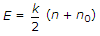Civil Engineering - Elements of Remote Sensing - Discussion

Discussion :: Elements of Remote Sensing - Section 3 (Q.No.7)

7.

Assuming n as the frequency of radiation, n0 as the threshold frequency and k as a constant, the energy of the photo-electrons (E) is related as

 [A]. E = k (n - n0) [B]. E = k (n + n0) [C]. E = k (n x n0) [D].Explanation:

No answer description available for this question.